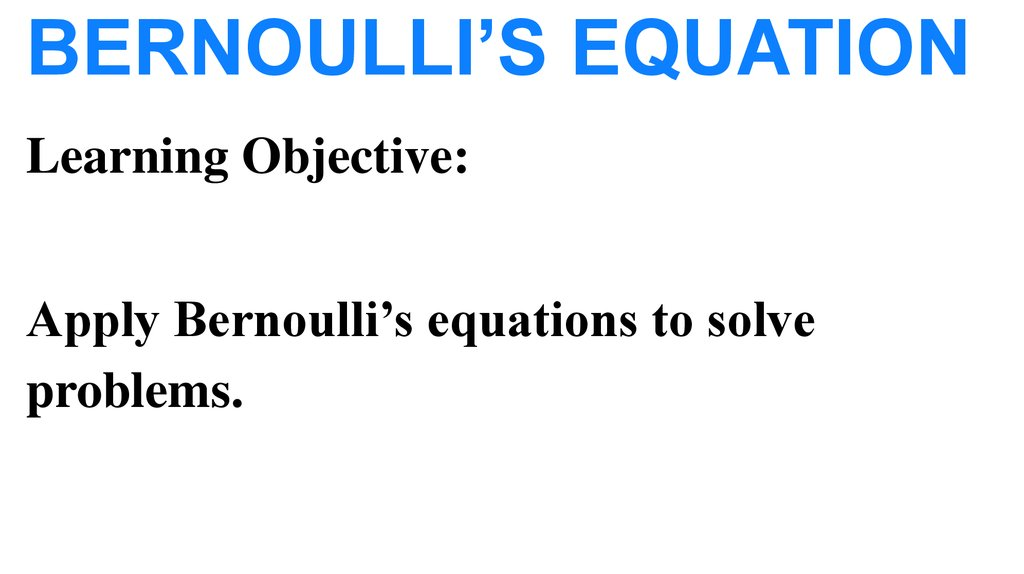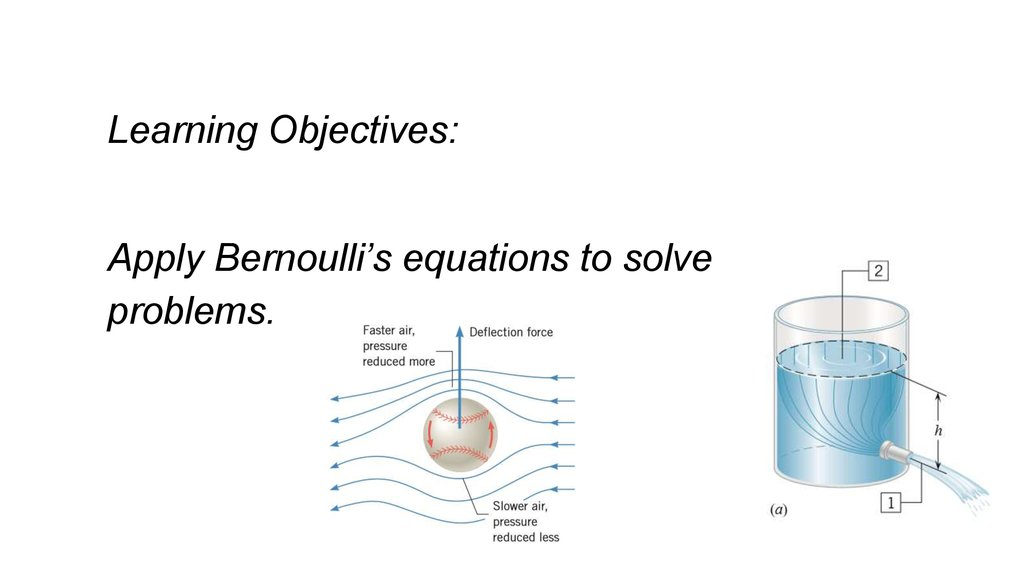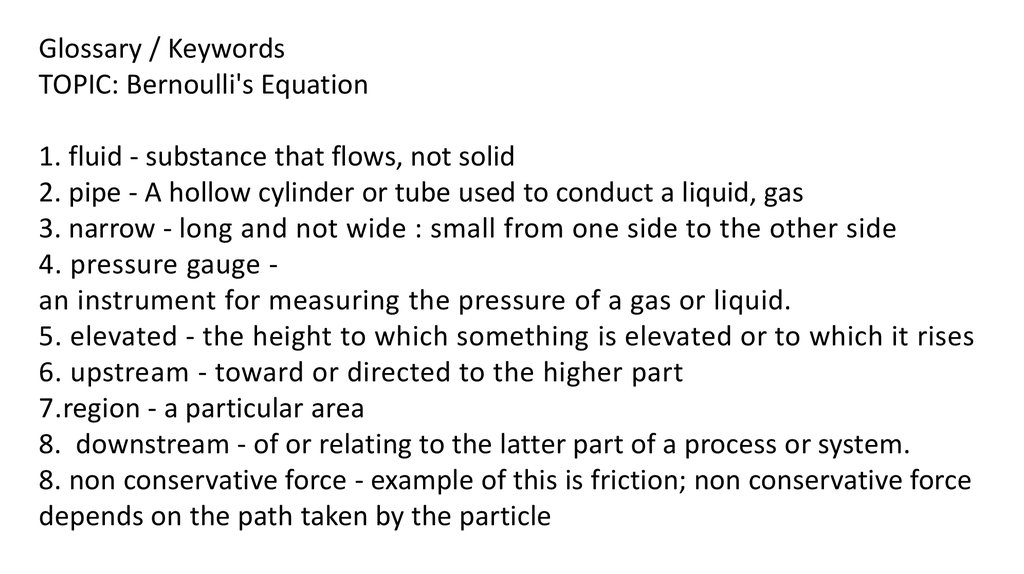# Bernoulli’s equation

## 1.

BERNOULLI’S EQUATION
Learning Objective:
Apply Bernoulli’s equations to solve
problems.

## 2.

Learning Objectives:
Apply Bernoulli’s equations to solve
problems.

## 3.

Glossary / Keywords
TOPIC: Bernoulli's Equation
1. fluid - substance that flows, not solid
2. pipe - A hollow cylinder or tube used to conduct a liquid, gas
3. narrow - long and not wide : small from one side to the other side
4. pressure gauge an instrument for measuring the pressure of a gas or liquid.
5. elevated - the height to which something is elevated or to which it rises
6. upstream - toward or directed to the higher part
7.region - a particular area
8. downstream - of or relating to the latter part of a process or system.
8. non conservative force - example of this is friction; non conservative force
depends on the path taken by the particle

## 4.

Whenever a fluid
is flowing in a
horizontal pipe and
encounters a region
of reduced crosssectional area, the
pressure
of the fluid drops, as
the pressure gauges
indicate.
First Observation

## 5.

When moving from
the wider region 2 to
the narrower region
1, the fluid speeds up
or accelerates,
consistent with the
conservation of mass
(as expressed by
the equation of
continuity).

## 6.

Second Observation
If the fluid
moves to a
higher
elevation,
the pressure at
the lower level
is greater than
the pressure at
the higher level.

## 7.

Ley us have another situation..
This drawing shows a
fluid element
of mass m, upstream in
region 2 of a pipe. Both
the cross-sectional area
and the elevation
are different at different
places along the pipe.

## 8.

The speed, pressure,
and elevation in this
region are v2, P2, and
y2, respectively.
Downstream in
region 1 these
variables have the
values
v1, P1, and y1.

## 9.

• Recall that from the Law of Conservation of
MECHANICAL ENERGY, an object moving under
the influence of gravity has a total mechanical
energy E that is the sum of the kinetic energy KE
and the gravitational potential energy PE:
2
E = KE + PE 1/2mv + mgy

## 10.

When work Wnc is done on the fluid element by
external nonconservative forces, the total mechanical
energy changes. According to the work–energy
theorem, the work equals the change in the total
mechanical energy:

## 11.

This figure shows how the
work Wnc arises. On the
top surface of the fluid
element, the surrounding
fluid exerts a pressure P.
This pressure gives rise to
a force of magnitude F
PA, where A is the crosssectional area.

## 12.

On the bottom surface,
the surrounding fluid
exerts a slightly greater
pressure, P + ΔP, where
ΔP is the pressure
difference between the
ends of the element.

## 13.

As a result, the force on the
bottom surface has a
magnitude of
F + ΔF = (P + ΔP)A.
The magnitude of the net
force pushing the
fluid element up the pipe is
Δ F = (ΔP)A.

## 14.

When the fluid element
moves through its own
length s, the work done
is the product of the
magnitude of the net
force and the distance:
Work = (ΔF)s = (ΔP)As.

## 15.

The quantity As is the
volume V of the element, so
the work is (ΔP)V. The total
work done on the fluid
element in moving it from
region 2 to region 1 is the
sum of the small increments
of work (ΔP)V done as the
element moves along the
pipe.

## 16.

This sum amounts to
Wnc = (P2 - P1)V,
where P2 - P1 is the
pressure difference
between the two regions.
With this expression for
Wnc, the work–energy
theorem becomes

## 17.

By dividing both sides of this result by the volume V,
recognizing that m/V is the density ρ of the fluid,
and rearranging terms, we obtain Bernoulli’s
equation.

## 18.

BERNOULLI’S EQUATION

## 19.

Since the points 1 and 2 were selected arbitrarily, the
term
has a constant value at all
positions in the flow. For this reason, Bernoulli’s
equation is sometimes expressed as

## 20.

When a moving fluid is contained in a horizontal
pipe, all parts of it have the same elevation (y1 = y2),
and Bernoulli’s equation simplifies to
Thus, the quantity
remains constant
throughout a horizontal pipe; if v increases,
P decreases, and vice versa.

## 21.

Example
An Enlarged Blood Vessel
An aneurysm is an abnormal enlargement
of a blood vessel such as the aorta. Because of an
aneurysm, the cross-sectional area A1 of the aorta
increases to a value of A2 = 1.7 A1. The speed of the
blood (ρ = 1060 kg/m3 ) through a normal portion of
the aorta is v1 = 0.40 m/s. Assuming that the aorta is
horizontal (the person is lying down), determine the
amount by which the pressure P2 in the enlarged
region exceeds the pressure P1 in the normal region.

## 22.

This equation may be used to nd
the pressure difference between two points in a uid
moving horizontally. However, in order to use this
relation we need to know the speed of the blood in
the enlarged region of the artery, as well as the speed
in the normal section. We can obtain the speed in the
enlarged region by using the equation of continuity
which relates it to the speed in the normal region and
the cross-sectional areas of the two parts.

## 26.

PRACTICAL EXAMPLE: The physics of household plumbing.
The impact of uid ow on pressure is widespread. Figure
below illustrates how household plumbing takes into
account the implications of Bernoulli’s equation. The Ushaped section of pipe beneath the sink is called a “trap,”
because it traps water, which serves as a barrier to prevent
sewer gas from leaking into the
house.

## 27.

Part a of the drawing shows poor plumbing. When water from
the clothes washer rushes through the sewer pipe, the highspeed ow causes the pressure at point A to drop. The pressure
at point B in the sink, however, remains at the higher
atmospheric pressure. As a result of this pressure difference,
the water is pushed out of the trap and into the sewer line,
leaving no protection against sewer gas.
Part
a
poor plumbing

## 28.

A correctly designed system is vented
to the outside of the house. The vent
ensures that the pressure at A remains
the same as that at B (atmospheric
pressure), even when water
from the clothes washer is
rushing through the pipe.
Thus, the purpose of the vent
is to prevent the trap from
being emptied, not to
provide an escape route
for sewer gas.